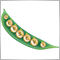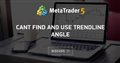# (iBands(upper)-iBands(lower))/iBands(lower) can't be plotted313

I could not get bbwidthBuffer[i] drawn by the terminal. It would show a blank indicator window beneath the price chart. What is wrong with my coding?

```Counted_bars=IndicatorCounted();
i=Bars-Counted_bars-1;
while(i>=0)
{
bbwidthBuffer[i]=(iBands(NULL,PERIOD_CURRENT,bb_period,2,0,PRICE_CLOSE,MODE_UPPER,i)-iBands(NULL,PERIOD_CURRENT,bb_period,2,0,PRICE_CLOSE,MODE_LOWER,i))/iBands(NULL,PERIOD_CURRENT,bb_period,2,0,PRICE_CLOSE,MODE_LOWER,i);
i--;
}```6660

You have to check for zero divide errors.

```//+------------------------------------------------------------------+
//| Custom indicator iteration function                              |
//+------------------------------------------------------------------+
int OnCalculate(const int rates_total,
const int prev_calculated,
const datetime &time[],
const double &open[],
const double &high[],
const double &low[],
const double &close[],
const long &tick_volume[],
const long &volume[],
{
//---
int begin=!prev_calculated?rates_total-BBandPeriod-1:rates_total-prev_calculated;
double bandsUpper,bandsLower;
//---
for(int i=begin;i>=0;i--)
{
bandsUpper=iBands(NULL,PERIOD_CURRENT,BBandPeriod,2,0,PRICE_CLOSE,MODE_UPPER,i);
bandsLower=iBands(NULL,PERIOD_CURRENT,BBandPeriod,2,0,PRICE_CLOSE,MODE_LOWER,i);
BBandsWidthBuffer[i]=(bandsLower)?(bandsUpper-bandsLower)/bandsLower:BBandsWidthBuffer[i+1];
}
//--- return value of prev_calculated for next call
return(rates_total);
}
//+------------------------------------------------------------------+```313

Ernst Van Der Merwe:

You have to check for zero divide errors.

Thank you very much Ernst for shedding on light on me as a newbie in mql4 programming.

Mind if I ask about the topic of how to normalize data given by iBands(upper) - iBands(lower) so that a peak will correspond to 100% and a trough to 0%?

Or is it easier to program code to detect a peak/trough formed and turning around by level / tangent/ gradient?6660

Wilson Wong:

Thank you very much Ernst for shedding on light on me as a newbie in mql4 programming.

Mind if I ask about the topic of how to normalize data given by iBands(upper) - iBands(lower) so that a peak will correspond to 100% and a trough to 0%?

Or is it easier to program code to detect a peak/trough formed and turning around by level / tangent/ gradient?

You're welcome. The easiest way would probably be to apply RSI.

```//--- indicator buffers
double         RSIBuffer[];
double         BBandsWidthBuffer[];
//+------------------------------------------------------------------+
//| Custom indicator initialization function                         |
//+------------------------------------------------------------------+
int OnInit()
{
//--- indicator buffers mapping
SetIndexBuffer(0,RSIBuffer);
IndicatorBuffers(2);
SetIndexBuffer(1,BBandsWidthBuffer);
//---
return(INIT_SUCCEEDED);
}
//+------------------------------------------------------------------+
//| Custom indicator iteration function                              |
//+------------------------------------------------------------------+
int OnCalculate(const int rates_total,
const int prev_calculated,
const datetime &time[],
const double &open[],
const double &high[],
const double &low[],
const double &close[],
const long &tick_volume[],
const long &volume[],
{
//---
int begin=!prev_calculated?rates_total-BBandPeriod-1:rates_total-prev_calculated;
double bandsUpper,bandsLower;
//---
for(int i=begin;i>=0;i--)
{
bandsUpper=iBands(NULL,PERIOD_CURRENT,BBandPeriod,2,0,PRICE_CLOSE,MODE_UPPER,i);
bandsLower=iBands(NULL,PERIOD_CURRENT,BBandPeriod,2,0,PRICE_CLOSE,MODE_LOWER,i);
BBandsWidthBuffer[i]=(bandsLower)?(bandsUpper-bandsLower)/bandsLower:BBandsWidthBuffer[i+1];
}
//---
begin=!prev_calculated?rates_total-BBandPeriod*2-2:rates_total-prev_calculated;
//---
for(int i=begin;i>=0;i--)
RSIBuffer[i]=iRSIOnArray(BBandsWidthBuffer,0,BBandPeriod,i);
//--- return value of prev_calculated for next call
return(rates_total);
}
//+------------------------------------------------------------------+
```313

Ernst Van Der Merwe:

You're welcome. The easiest way would probably be to apply RSI.

RSIBuffer on BBandsWidthBuffer did not quite work. The value grows eventually quite large and offers not much of useful indication.

I have found another thread about calculating the slope/angle of price/indicator at

https://www.mql5.com/en/forum/155802Cant find and use trendline angle
• 2015.06.06
• www.mql5.com
What I want to do is to create a trendline with angle based on 2 anchor points and then find the angle using ObjectGetDouble() so it can help me do...